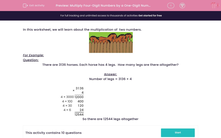# Multiply Four-Digit Numbers by a One-Digit Number

In this worksheet, student use a column method to multiply four-digit numbers by a one-digit numberThis content is premium and exclusive to EdPlace subscribers.Key stage:  KS 2

Curriculum topic:   Maths and Numerical Reasoning

Curriculum subtopic:   Mixed Problems

Difficulty level:#### Worksheet Overview

In this worksheet, we will learn about the multiplication of  two numbers.For Example:

Question:

There are 3136 horses. Each horse has 4 legs.  How many legs are there altogether?

Number of legs = 3136 × 4

 × 3 1 3 6 4 4 × 3000 1 2 0 0 0 4 × 100 4 0 0 4 × 30 1 2 0 4 × 6 2 4 1 2 5 4 4

So there are 12544 legs altogether

### What is EdPlace?

We're your National Curriculum aligned online education content provider helping each child succeed in English, maths and science from year 1 to GCSE. With an EdPlace account you’ll be able to track and measure progress, helping each child achieve their best. We build confidence and attainment by personalising each child’s learning at a level that suits them.

Get started••••